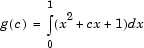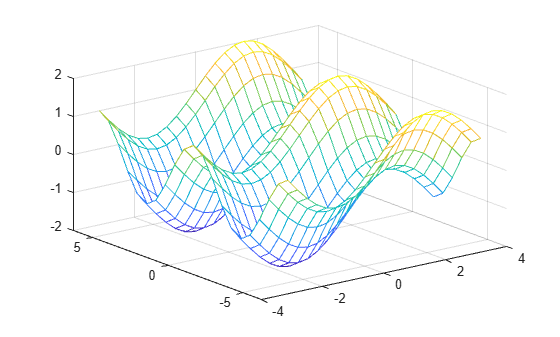## Anonymous Functions

### What Are Anonymous Functions?

An anonymous function is a function that is not stored in a program file, but is associated with a variable whose data type is `function_handle`. Anonymous functions can accept multiple inputs and return one output. They can contain only a single executable statement.

For example, create a handle to an anonymous function that finds the square of a number:

`sqr = @(x) x.^2;`

Variable `sqr` is a function handle. The `@` operator creates the handle, and the parentheses `()` immediately after the `@` operator include the function input arguments. This anonymous function accepts a single input `x`, and implicitly returns a single output, an array the same size as `x` that contains the squared values.

Find the square of a particular value (`5`) by passing the value to the function handle, just as you would pass an input argument to a standard function.

`a = sqr(5)`
```a = 25```

Many MATLAB® functions accept function handles as inputs so that you can evaluate functions over a range of values. You can create handles either for anonymous functions or for functions in program files. The benefit of using anonymous functions is that you do not have to edit and maintain a file for a function that requires only a brief definition.

For example, find the integral of the `sqr` function from `0` to `1` by passing the function handle to the `integral` function:

`q = integral(sqr,0,1);`

You do not need to create a variable in the workspace to store an anonymous function. Instead, you can create a temporary function handle within an expression, such as this call to the `integral` function:

`q = integral(@(x) x.^2,0,1);`

### Variables in the Expression

Function handles can store not only an expression, but also variables that the expression requires for evaluation.

For example, create a handle to an anonymous function that requires coefficients `a`, `b`, and `c`.

```a = 1.3; b = .2; c = 30; parabola = @(x) a*x.^2 + b*x + c;```

Because `a`, `b`, and `c` are available at the time you create `parabola`, the function handle includes those values. The values persist within the function handle even if you clear the variables:

```clear a b c x = 1; y = parabola(x)```
```y = 31.5000```

To supply different values for the coefficients, you must create a new function handle:

```a = -3.9; b = 52; c = 0; parabola = @(x) a*x.^2 + b*x + c; x = 1; y = parabola(1)```
```y = 48.1000```

You can save function handles and their associated values in a MAT-file and load them in a subsequent MATLAB session using the `save` and `load` functions, such as

`save myfile.mat parabola`

Use only explicit variables when constructing anonymous functions. If an anonymous function accesses any variable or nested function that is not explicitly referenced in the argument list or body, MATLAB throws an error when you invoke the function. Implicit variables and function calls are often encountered in the functions such as `eval``evalin`, `assignin`, and `load`.  Avoid using these functions in the body of anonymous functions.

### Multiple Anonymous Functions

The expression in an anonymous function can include another anonymous function. This is useful for passing different parameters to a function that you are evaluating over a range of values. For example, you can solve the equationfor varying values of `c` by combining two anonymous functions:

`g = @(c) (integral(@(x) (x.^2 + c*x + 1),0,1));`

Here is how to derive this statement:

1. Write the integrand as an anonymous function,

`@(x) (x.^2 + c*x + 1)`
2. Evaluate the function from zero to one by passing the function handle to `integral`,

`integral(@(x) (x.^2 + c*x + 1),0,1)`
3. Supply the value for `c` by constructing an anonymous function for the entire equation,

`g = @(c) (integral(@(x) (x.^2 + c*x + 1),0,1));`

The final function allows you to solve the equation for any value of `c`. For example:

`g(2)`
```ans = 2.3333```

### Functions with No Inputs

If your function does not require any inputs, use empty parentheses when you define and call the anonymous function. For example:

```t = @() datestr(now); d = t()```
```d = 26-Jan-2012 15:11:47```

Omitting the parentheses in the assignment statement creates another function handle, and does not execute the function:

`d = t`
```d = @() datestr(now)```

### Functions with Multiple Inputs or Outputs

Anonymous functions require that you explicitly specify the input arguments as you would for a standard function, separating multiple inputs with commas. For example, this function accepts two inputs, `x` and `y`:

```myfunction = @(x,y) (x^2 + y^2 + x*y); x = 1; y = 10; z = myfunction(x,y)```
```z = 111 ```

However, an anonymous function returns only one output. If the expression in the function returns multiple outputs, then you can request them when you invoke the function handle.

For example, the `ndgrid` function can return as many outputs as the number of input vectors. This anonymous function that calls `ndgrid` returns only one output (`mygrid`). Invoke `mygrid` to access the outputs returned by the `ndgrid` function.

```c = 10; mygrid = @(x,y) ndgrid((-x:x/c:x),(-y:y/c:y)); [x,y] = mygrid(pi,2*pi);```

You can use the output from `mygrid` to create a mesh or surface plot:

```z = sin(x) + cos(y); mesh(x,y,z)```### Arrays of Anonymous Functions

Although most MATLAB fundamental data types support multidimensional arrays, function handles must be scalars (single elements). However, you can store multiple function handles using a cell array or structure array. The most common approach is to use a cell array, such as

```f = {@(x)x.^2; @(y)y+10; @(x,y)x.^2+y+10};```

When you create the cell array, keep in mind that MATLAB interprets spaces as column separators. Either omit spaces from expressions, as shown in the previous code, or enclose expressions in parentheses, such as

```f = {@(x) (x.^2); @(y) (y + 10); @(x,y) (x.^2 + y + 10)};```

Access the contents of a cell using curly braces. For example, `f{1}` returns the first function handle. To execute the function, pass input values in parentheses after the curly braces:

```x = 1; y = 10; f{1}(x) f{2}(y) f{3}(x,y)```
```ans = 1 ans = 20 ans = 21```